# Counting Sort – Data Structures and Algorithms Tutorials

## What is Counting Sort?

Counting Sort is a non-comparison-based sorting algorithm that works well when there is limited range of input values. It is particularly efficient when the range of input values is small compared to the number of elements to be sorted. The basic idea behind Counting Sort is to count the frequency of each distinct element in the input array and use that information to place the elements in their correct sorted positions.

## How does Counting Sort Algorithm work?

Step1 :

• Find out the maximum element from the given array.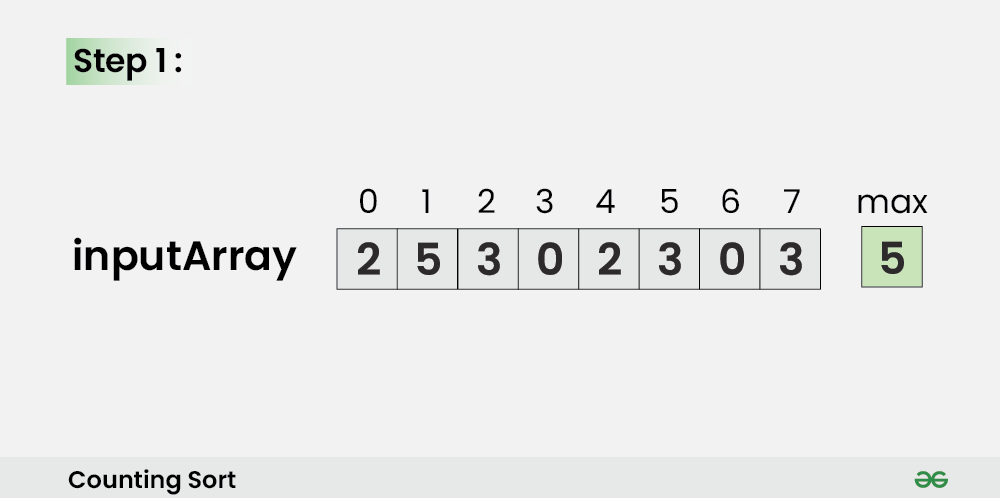Step 2:

• Initialize a countArray[] of length max+1 with all elements as 0. This array will be used for storing the occurrences of the elements of the input array.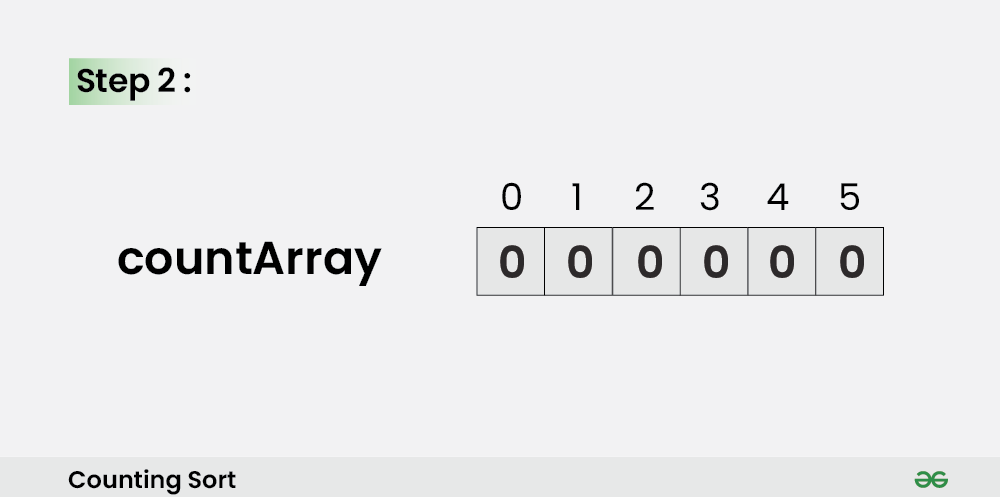Step 3:

• In the countArray[], store the count of each unique element of the input array at their respective indices.
• For Example: The count of element 2 in the input array is 2. So, store 2 at index 2 in the countArray[]. Similarly, the count of element 5 in the input array is 1, hence store 1 at index 5 in the countArray[].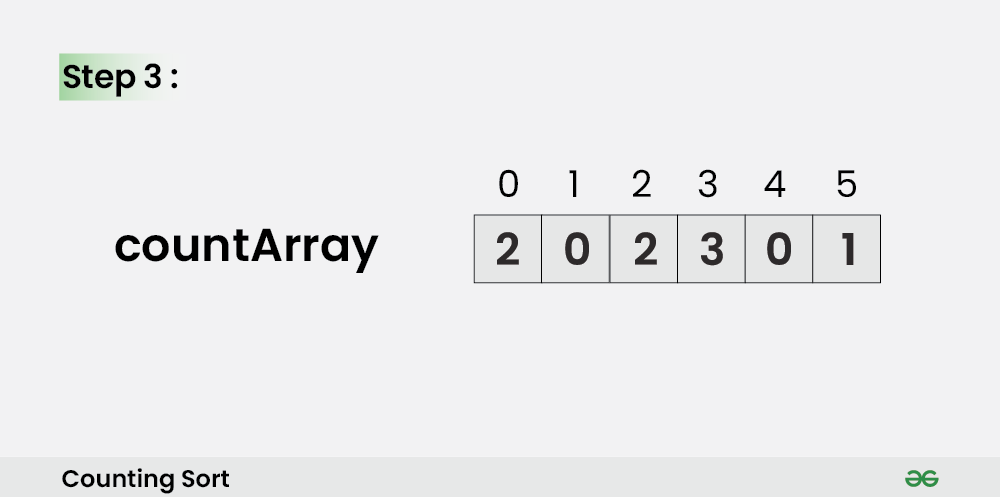Step 4:

• Store the cumulative sum or prefix sum of the elements of the countArray[] by doing countArray[i] = countArray[i – 1] + countArray[i]. This will help in placing the elements of the input array at the correct index in the output array.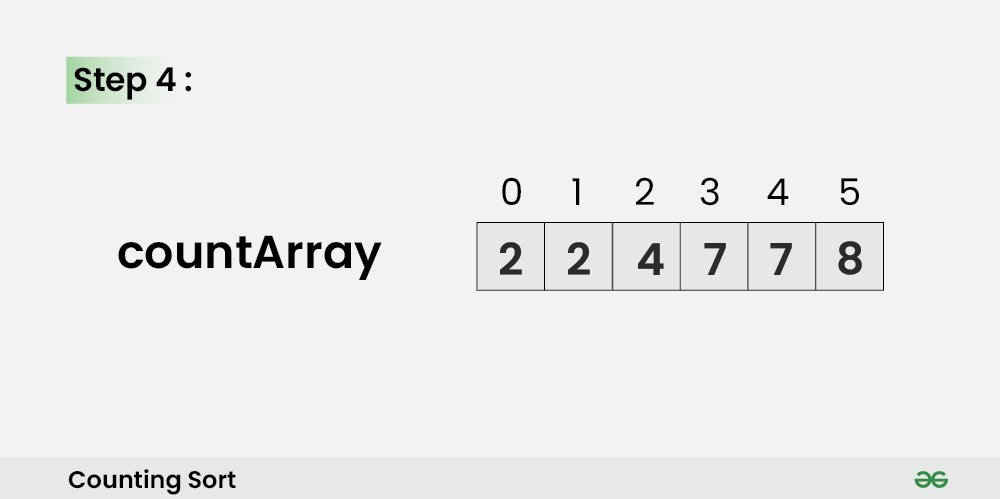Step 5:

• Iterate from end of the input array and because traversing input array from end preserves the order of equal elements, which eventually makes this sorting algorithm stable.
• Update outputArray[ countArray[ inputArray[i] ] – 1] = inputArray[i].
• Also, update countArray[ inputArray[i] ] = countArray[ inputArray[i] ]– -.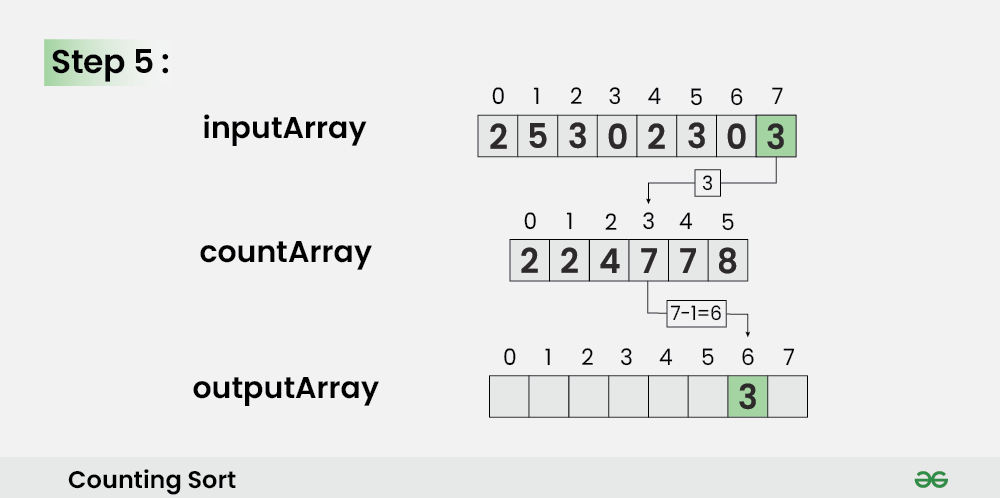Step 6: For i = 6,

Update outputArray[ countArray[ inputArray ] – 1] = inputArray
Also, update countArray[ inputArray ] = countArray[ inputArray ]- –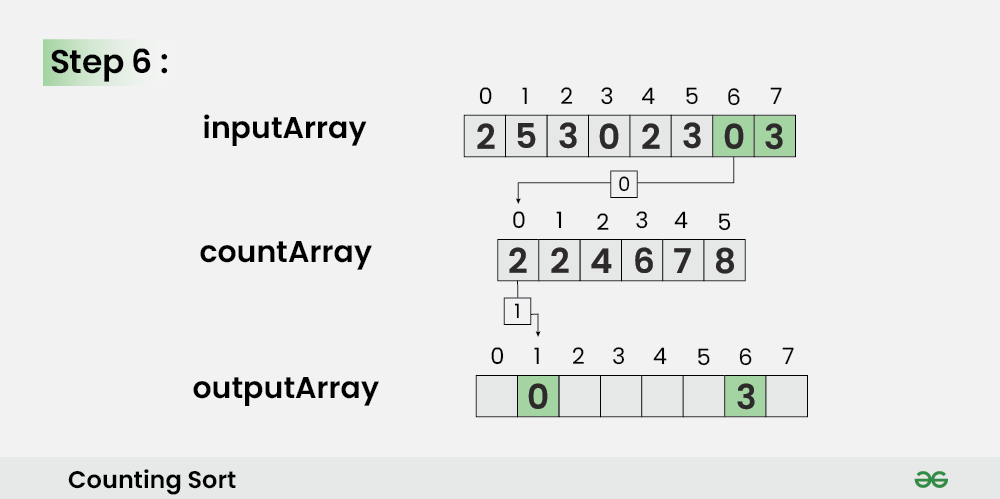Step 7: For i = 5,

Update outputArray[ countArray[ inputArray ] – 1] = inputArray
Also, update countArray[ inputArray ] = countArray[ inputArray ]- –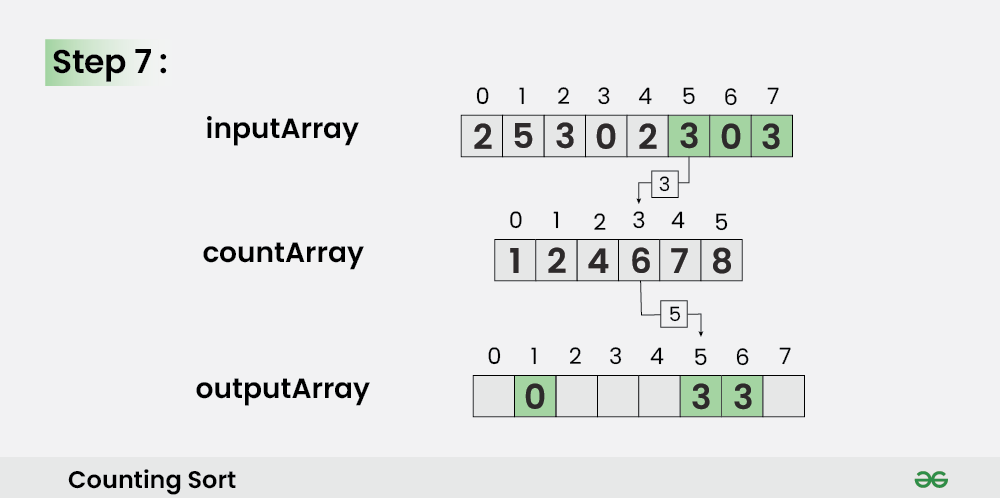Step 8: For i = 4,

Update outputArray[ countArray[ inputArray ] – 1] = inputArray
Also, update countArray[ inputArray ] = countArray[ inputArray ]- –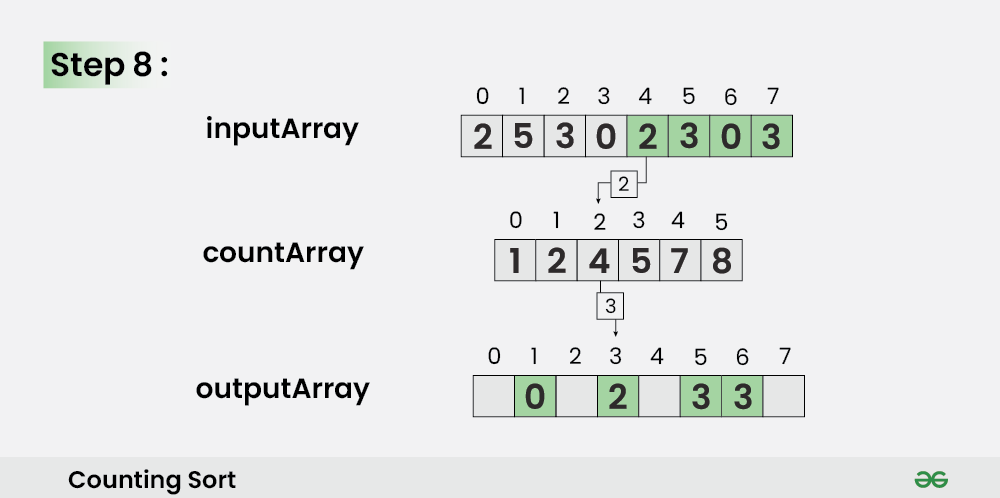Step 9: For i = 3,

Update outputArray[ countArray[ inputArray ] – 1] = inputArray
Also, update countArray[ inputArray ] = countArray[ inputArray ]- –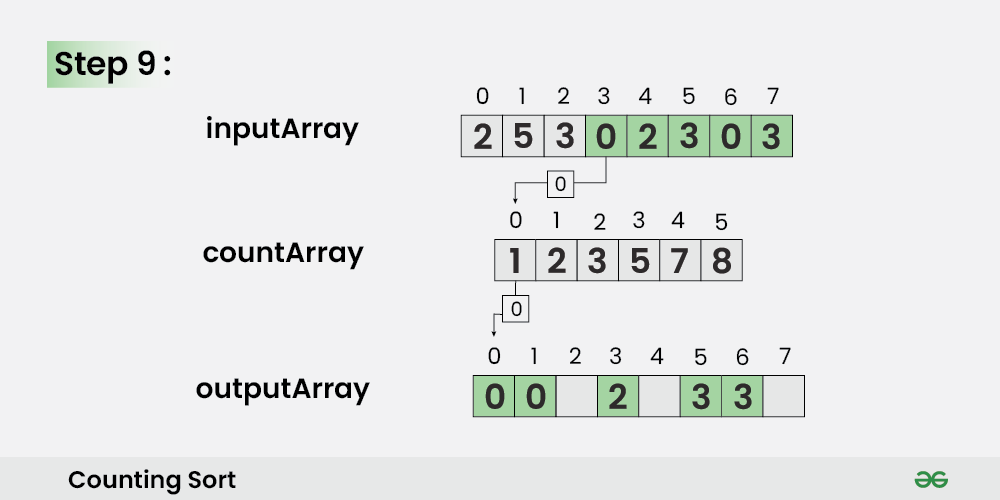Step 10: For i = 2,

Update outputArray[ countArray[ inputArray ] – 1] = inputArray
Also, update countArray[ inputArray ] = countArray[ inputArray ]- –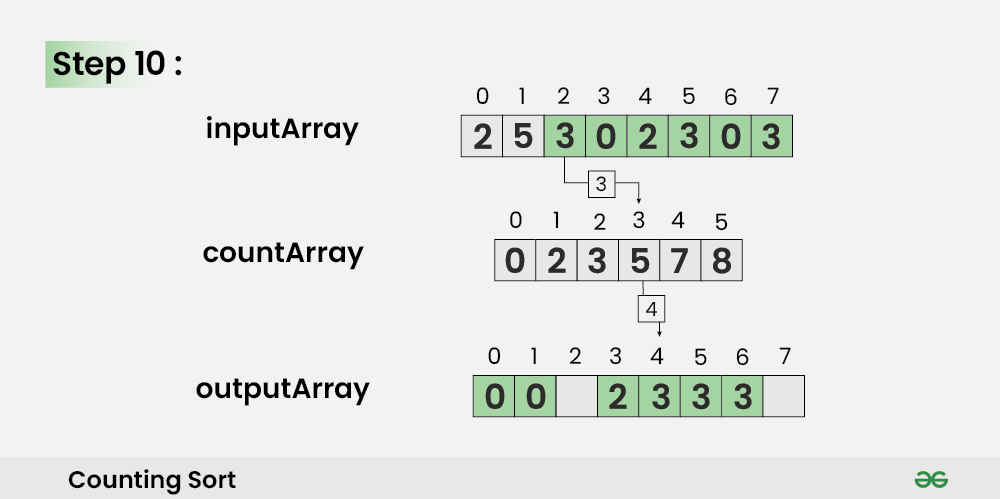Step 11: For i = 1,

Update outputArray[ countArray[ inputArray ] – 1] = inputArray
Also, update countArray[ inputArray ] = countArray[ inputArray ]- –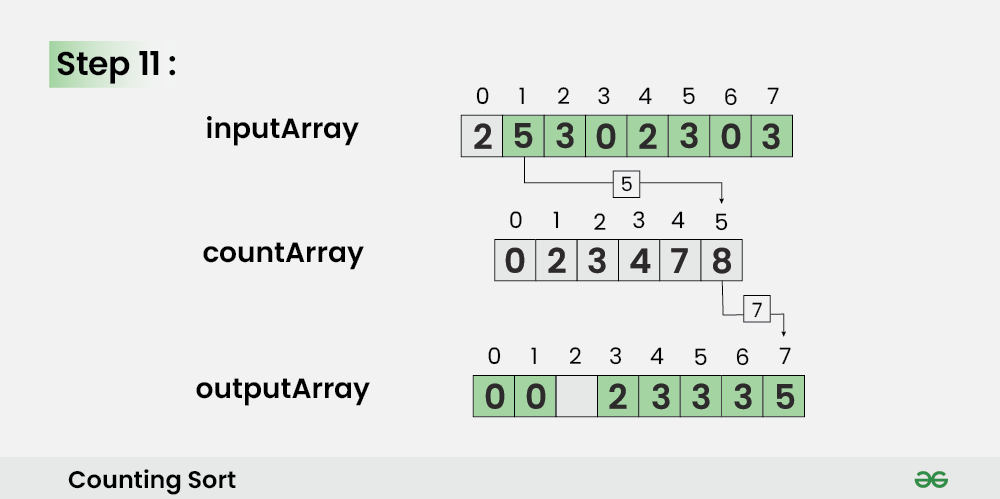Step 12: For i = 0,

Update outputArray[ countArray[ inputArray ] – 1] = inputArray
Also, update countArray[ inputArray ] = countArray[ inputArray ]- –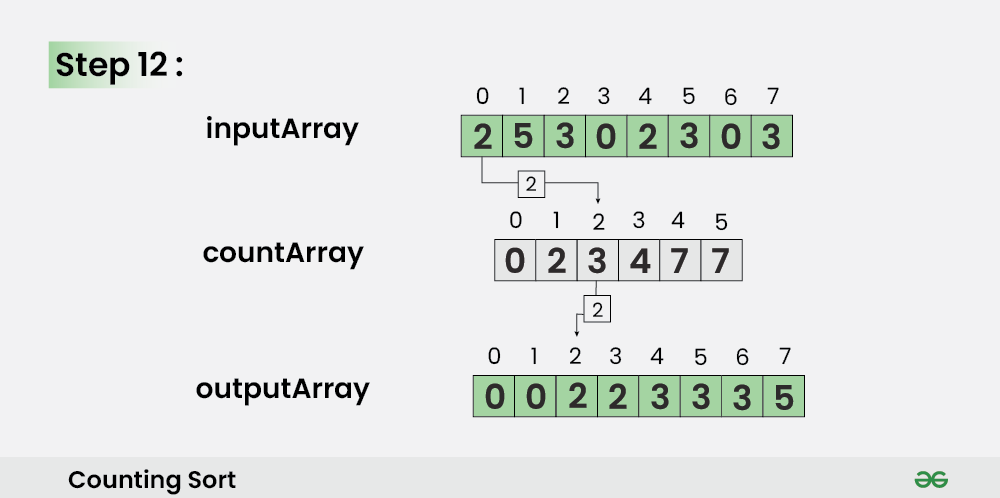## Counting Sort Algorithm:

• Declare an auxiliary array countArray[] of size max(inputArray[])+1 and initialize it with 0.
• Traverse array inputArray[] and map each element of inputArray[] as an index of countArray[] array, i.e., execute countArray[inputArray[i]]++ for 0 <= i < N.
• Calculate the prefix sum at every index of array inputArray[].
• Create an array outputArray[] of size N.
• Traverse array inputArray[] from end and update outputArray[ countArray[ inputArray[i] ] – 1] = inputArray[i]. Also, update countArray[ inputArray[i] ] = countArray[ inputArray[i] ]- – .

Below is the implementation of the above algorithm:

## C++14

 `#include ` `using` `namespace` `std;`   `vector<``int``> countSort(vector<``int``>& inputArray)` `{`   `    ``int` `N = inputArray.size();`   `    ``// Finding the maximum element of array inputArray[].` `    ``int` `M = 0;`   `    ``for` `(``int` `i = 0; i < N; i++)` `        ``M = max(M, inputArray[i]);`   `    ``// Initializing countArray[] with 0` `    ``vector<``int``> countArray(M + 1, 0);`   `    ``// Mapping each element of inputArray[] as an index` `    ``// of countArray[] array`   `    ``for` `(``int` `i = 0; i < N; i++)` `        ``countArray[inputArray[i]]++;`   `    ``// Calculating prefix sum at every index` `    ``// of array countArray[]` `    ``for` `(``int` `i = 1; i <= M; i++)` `        ``countArray[i] += countArray[i - 1];`   `    ``// Creating outputArray[] from countArray[] array` `    ``vector<``int``> outputArray(N);`   `    ``for` `(``int` `i = N - 1; i >= 0; i--)`   `    ``{` `        ``outputArray[countArray[inputArray[i]] - 1]` `            ``= inputArray[i];`   `        ``countArray[inputArray[i]]--;` `    ``}`   `    ``return` `outputArray;` `}`   `// Driver code` `int` `main()`   `{`   `    ``// Input array` `    ``vector<``int``> inputArray = { 4, 3, 12, 1, 5, 5, 3, 9 };`   `    ``// Output array` `    ``vector<``int``> outputArray = countSort(inputArray);`   `    ``for` `(``int` `i = 0; i < inputArray.size(); i++)` `        ``cout << outputArray[i] << ``" "``;`   `    ``return` `0;` `}`

## Java

 `import` `java.util.Arrays;`   `public` `class` `CountSort {` `    ``public` `static` `int``[] countSort(``int``[] inputArray) {` `        ``int` `N = inputArray.length;` `        ``int` `M = ``0``;`   `        ``for` `(``int` `i = ``0``; i < N; i++) {` `            ``M = Math.max(M, inputArray[i]);` `        ``}`   `        ``int``[] countArray = ``new` `int``[M + ``1``];`   `        ``for` `(``int` `i = ``0``; i < N; i++) {` `            ``countArray[inputArray[i]]++;` `        ``}`   `        ``for` `(``int` `i = ``1``; i <= M; i++) {` `            ``countArray[i] += countArray[i - ``1``];` `        ``}`   `        ``int``[] outputArray = ``new` `int``[N];`   `        ``for` `(``int` `i = N - ``1``; i >= ``0``; i--) {` `            ``outputArray[countArray[inputArray[i]] - ``1``] = inputArray[i];` `            ``countArray[inputArray[i]]--;` `        ``}`   `        ``return` `outputArray;` `    ``}`   `    ``public` `static` `void` `main(String[] args) {` `        ``int``[] inputArray = {``4``, ``3``, ``12``, ``1``, ``5``, ``5``, ``3``, ``9``};` `        ``int``[] outputArray = countSort(inputArray);`   `        ``for` `(``int` `i = ``0``; i < inputArray.length; i++) {` `            ``System.out.print(outputArray[i] + ``" "``);` `        ``}` `    ``}` `}`

## Python3

 `def` `count_sort(input_array):` `    ``# Finding the maximum element of input_array.` `    ``M ``=` `max``(input_array)`   `    ``# Initializing count_array with 0` `    ``count_array ``=` `[``0``] ``*` `(M ``+` `1``)`   `    ``# Mapping each element of input_array as an index of count_array` `    ``for` `num ``in` `input_array:` `        ``count_array[num] ``+``=` `1`   `    ``# Calculating prefix sum at every index of count_array` `    ``for` `i ``in` `range``(``1``, M ``+` `1``):` `        ``count_array[i] ``+``=` `count_array[i ``-` `1``]`   `    ``# Creating output_array from count_array` `    ``output_array ``=` `[``0``] ``*` `len``(input_array)`   `    ``for` `i ``in` `range``(``len``(input_array) ``-` `1``, ``-``1``, ``-``1``):` `        ``output_array[count_array[input_array[i]] ``-` `1``] ``=` `input_array[i]` `        ``count_array[input_array[i]] ``-``=` `1`   `    ``return` `output_array`   `# Driver code` `if` `__name__ ``=``=` `"__main__"``:` `    ``# Input array` `    ``input_array ``=` `[``4``, ``3``, ``12``, ``1``, ``5``, ``5``, ``3``, ``9``]`   `    ``# Output array` `    ``output_array ``=` `count_sort(input_array)`   `    ``for` `num ``in` `output_array:` `        ``print``(num, end``=``" "``)`

Output

`1 3 3 4 5 5 9 12 `

## Complexity Analysis of Counting Sort:

• Time Complexity: O(N+M), where N and M are the size of inputArray[] and countArray[] respectively.
• Worst-case: O(N+M).
• Average-case: O(N+M).
• Best-case: O(N+M).
• Auxiliary Space: O(N+M), where N and M are the space taken by outputArray[] and countArray[] respectively.

• Counting sort generally performs faster than all comparison-based sorting algorithms, such as merge sort and quicksort, if the range of input is of the order of the number of input.
• Counting sort is easy to code
• Counting sort is a stable algorithm.